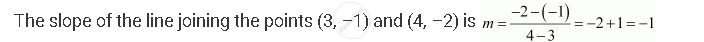# Find the Angle Between the X-axis and the Line Joining the Points (3, –1) and (4, –2). - Mathematics

Find the angle between the x-axis and the line joining the points (3, –1) and (4, –2).

#### SolutionNow, the inclination (θ ) of the line joining the points (3, –1) and (4, – 2) is given by

tan θ= –1

⇒ θ = (90° + 45°) = 135°

Thus, the angle between the x-axis and the line joining the points (3, –1) and (4, –2) is 135°.

Concept: Slope of a Line
Is there an error in this question or solution?

#### APPEARS IN

NCERT Class 11 Mathematics
Chapter 10 Straight Lines
Q 10 | Page 212